• 图像处理中的深度学习

# 什么是深度学习？

• 它是 ML 的子集。
• 它使用多层（非线性）处理单元级联，称为人工神经网络（ANN），以及受大脑（神经元）结构和功能启发的算法。每个连续层使用前一层的输出作为输入。
• 它使用人工神经网络进行特征提取和转换，处理数据，发现模式，并开发抽象。
• 它可以是有监督的（例如，分类）或无监督的（例如，模式分析）。
• 它使用梯度下降算法来学习对应于不同抽象级别的多个表示级别，以及。。。

# 经典与深度学习

• 手工与自动特征提取：为了解决传统 ML 技术的图像处理问题，最重要的预处理步骤是手工特征（如 HOG 和 SIFT）提取，以降低图像的复杂性，并使模式更加可见，以便学习算法工作。深度学习算法的最大优点是，它们试图以增量方式从训练图像中学习低级和高级特征。这样就不需要在提取或工程中使用手工制作的功能。
• 分部分与端到端解决方案：传统的 ML 技术通过分解问题、先解决不同部分，然后将结果聚合到一起给出输出来解决问题陈述，而深度学习技术使用端到端方法解决问题。例如，在对象检测问题中，经典的 ML 算法（如 SVM）需要一个边界框对象检测算法，该算法将首先识别所有可能的对象，这些对象需要将 HOG 作为 ML 算法的输入，以便识别正确的对象。但一种深度学习方法，如 YOLO 网络，将图像作为输入，并提供对象的位置和名称作为输出。显然是端到端的，不是吗？
• 训练时间和先进的硬件：与传统的 ML 算法不同，深度学习算法由于参数数量庞大、数据集相对庞大，训练时间较长。因此，我们应该始终在高端硬件（如 GPU）上训练深度学习模型，并记住合理的训练时间，因为时间是有效训练模型的一个非常重要的方面。

• 适应性和可转移性经典的 ML 技术非常有限，而深度学习技术可以应用于广泛的应用和各个领域。其中很大一部分用于转移学习，这使我们能够在同一领域内为不同的应用程序使用预先训练的深度网络。例如，在这里，在图像处理中，预训练的图像分类网络通常用作特征提取前端来检测对象和分割网络。

**现在，让我们以图解方式（猫和狗的图像）在图像分类中使用 ML 和深度学习模型时，看看它们之间的区别。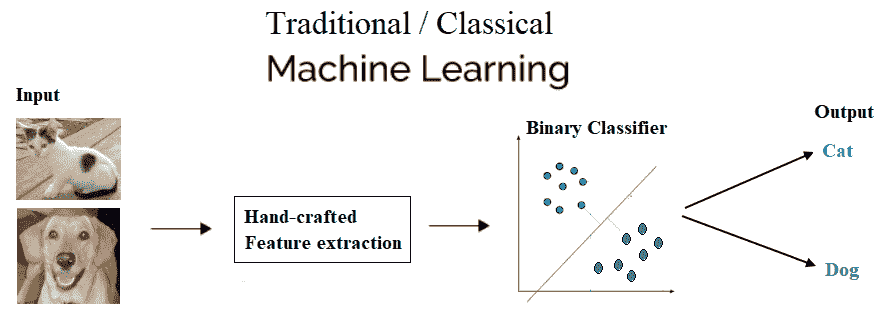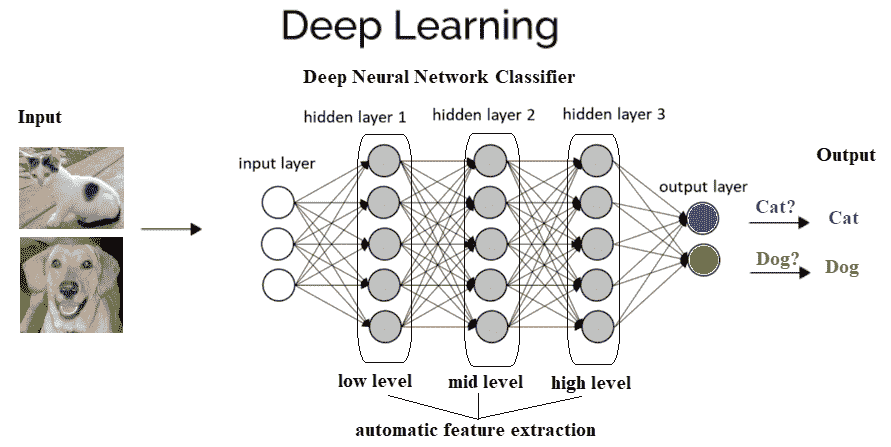# 为什么要深入学习？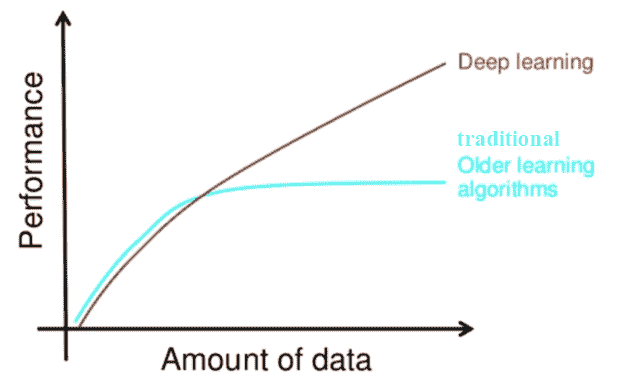# CNNs

CNN是深度神经网络，主要用于输入图像。CNN 学习传统算法中手工设计的滤波器（特征）。这种独立于先验知识和人类在特征设计中的努力是一个主要优势。它们还通过共享权重体系结构减少了需要学习的参数数量，并具有平移不变性特征。在下一小节中，我们将讨论 CNN 的总体架构及其工作原理。

# Conv 或池或 FC 层–CNN 体系结构及其工作原理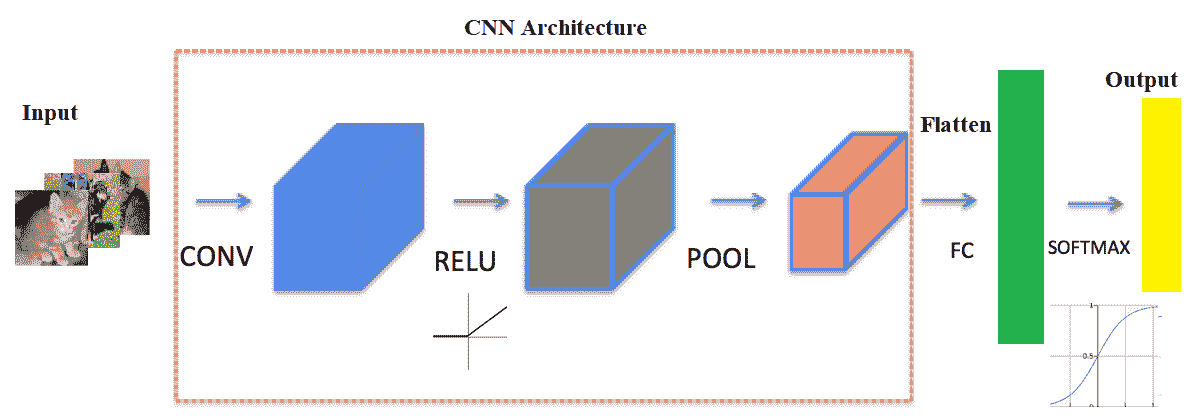# 卷积层

CNN 的主要组成部分是卷积层。卷积层由一组卷积滤波器（内核）组成，我们已经在第 2 章采样、傅里叶变换和卷积中详细讨论了这些滤波器。使用卷积滤波器对输入图像进行卷积，以生成特征映射。左侧是卷积层的输入；例如，输入图像。右边是卷积滤波器，也称为内核。通常，卷积运算是通过在输入上滑动该滤波器来执行的。在每个位置，元素矩阵乘法的和进入特征映射。卷积层由其宽度、高度（过滤器的大小为宽度 x 高度）和深度（过滤器数量）表示。步长指定卷积过滤器在每一步移动的量（默认值为 1）。填充是指环绕输入的零层（通常用于保持输入和输出图像大小相同，也称为相同填充。以下屏幕截图显示了如何在 RGB 图像上应用 3 x 3 x 3 卷积滤波器，第一个使用有效填充，第二个使用两个大小为步长=填充=1的此类滤波器进行计算：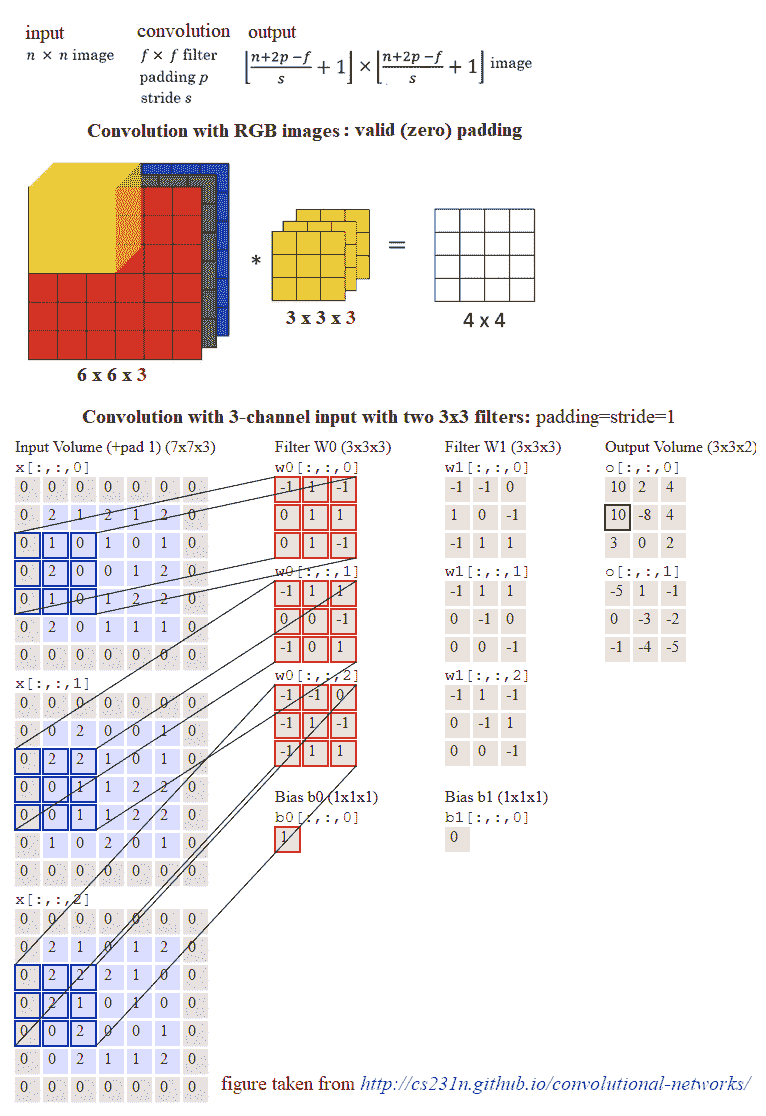# 辍学者

Dropout是深度神经网络最流行的正则化技术。Dropout 用于防止过度拟合，通常用于在看不见的数据集上提高深度学习任务的性能（准确性）。在训练期间，在每次迭代中，一个神经元以某种概率暂时丢失或禁用，p。这意味着在当前迭代中，该神经元的所有输入和输出都将被禁用。这个超参数p被称为退出率，它通常是一个 0.5 左右的数字，对应于 50%的神经元退出。

# 基于 TensorFlow 或 Keras 的图像分类

*# TF 分类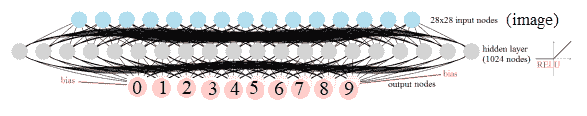%matplotlib inline
import numpy as np
# import data
from keras.datasets import mnist
import tensorflow as tf

(X_train, y_train), (X_test, y_test) = mnist.load_data()
np.random.seed(0)
train_indices = np.random.choice(60000, 50000, replace=False)
valid_indices = [i for i in range(60000) if i not in train_indices]
X_valid, y_valid = X_train[valid_indices,:,:], y_train[valid_indices]
X_train, y_train = X_train[train_indices,:,:], y_train[train_indices]
print(X_train.shape, X_valid.shape, X_test.shape)
# (50000, 28, 28) (10000, 28, 28) (10000, 28, 28)
image_size = 28
num_labels = 10

def reformat(dataset, labels):
dataset = dataset.reshape((-1, image_size * image_size)).astype(np.float32)
# one hot encoding: Map 1 to [0.0, 1.0, 0.0 ...], 2 to [0.0, 0.0, 1.0 ...]
labels = (np.arange(num_labels) == labels[:,None]).astype(np.float32)
return dataset, labels

X_train, y_train = reformat(X_train, y_train)
X_valid, y_valid = reformat(X_valid, y_valid)
X_test, y_test = reformat(X_test, y_test)
print('Training set', X_train.shape, X_train.shape)
print('Validation set', X_valid.shape, X_valid.shape)
print('Test set', X_test.shape, X_test.shape)
# Training set (50000, 784) (50000, 784) # Validation set (10000, 784) (10000, 784) # Test set (10000, 784) (10000, 784)

def accuracy(predictions, labels):
return (100.0 * np.sum(np.argmax(predictions, 1) == np.argmax(labels, 1)) / predictions.shape)

batch_size = 256
num_hidden_units = 1024
lambda1 = 0.1
lambda2 = 0.1

graph = tf.Graph()
with graph.as_default():

# Input data. For the training data, we use a placeholder that will be fed
# at run time with a training minibatch.
tf_train_dataset = tf.placeholder(tf.float32,
shape=(batch_size, image_size * image_size))
tf_train_labels = tf.placeholder(tf.float32, shape=(batch_size, num_labels))
tf_valid_dataset = tf.constant(X_valid)
tf_test_dataset = tf.constant(X_test)

# Variables.
weights1 = tf.Variable(tf.truncated_normal([image_size * image_size, num_hidden_units]))
biases1 = tf.Variable(tf.zeros([num_hidden_units]))

# connect inputs to every hidden unit. Add bias
layer_1_outputs = tf.nn.relu(tf.matmul(tf_train_dataset, weights1) + biases1)

weights2 = tf.Variable(tf.truncated_normal([num_hidden_units, num_labels]))
biases2 = tf.Variable(tf.zeros([num_labels]))

# Training computation.
logits = tf.matmul(layer_1_outputs, weights2) + biases2
loss = tf.reduce_mean(tf.nn.softmax_cross_entropy_with_logits(labels=tf_train_labels, logits=logits) + \
lambda1*tf.nn.l2_loss(weights1) + lambda2*tf.nn.l2_loss(weights2))

# Optimizer.

# Predictions for the training, validation, and test data.
train_prediction = tf.nn.softmax(logits)
layer_1_outputs = tf.nn.relu(tf.matmul(tf_valid_dataset, weights1) + biases1)
valid_prediction = tf.nn.softmax(tf.matmul(layer_1_outputs, weights2) + biases2)
layer_1_outputs = tf.nn.relu(tf.matmul(tf_test_dataset, weights1) + biases1)
test_prediction = tf.nn.softmax(tf.matmul(layer_1_outputs, weights2) + biases2)

num_steps = 6001

ll = []
atr = []
av = []

import matplotlib.pylab as pylab

with tf.Session(graph=graph) as session:
#tf.global_variables_initializer().run()
session.run(tf.initialize_all_variables())
print("Initialized")
for step in range(num_steps):
# Pick an offset within the training data, which has been randomized.
# Note: we could use better randomization across epochs.
offset = (step * batch_size) % (y_train.shape - batch_size)
# Generate a minibatch.
batch_data = X_train[offset:(offset + batch_size), :]
batch_labels = y_train[offset:(offset + batch_size), :]
# Prepare a dictionary telling the session where to feed the minibatch.
# The key of the dictionary is the placeholder node of the graph to be fed,
# and the value is the numpy array to feed to it.
feed_dict = {tf_train_dataset : batch_data, tf_train_labels : batch_labels}
_, l, predictions = session.run([optimizer, loss, train_prediction], feed_dict=feed_dict)
if (step % 500 == 0):
ll.append(l)
a = accuracy(predictions, batch_labels)
atr.append(a)
print("Minibatch loss at step %d: %f" % (step, l))
print("Minibatch accuracy: %.1f%%" % a)
a = accuracy(valid_prediction.eval(), y_valid)
av.append(a)
print("Validation accuracy: %.1f%%" % a)
print("Test accuracy: %.1f%%" % accuracy(test_prediction.eval(), y_test))

# Initialized
# Minibatch loss at step 0: 92091.781250
# Minibatch accuracy: 9.0%
# Validation accuracy: 21.6%
#
# Minibatch loss at step 500: 35599.835938
# Minibatch accuracy: 50.4%
# Validation accuracy: 47.4%
#
# Minibatch loss at step 1000: 15989.455078
# Minibatch accuracy: 46.5%
# Validation accuracy: 47.5%
#
# Minibatch loss at step 1500: 7182.631836
# Minibatch accuracy: 59.0%
# Validation accuracy: 54.7%
#
# Minibatch loss at step 2000: 3226.800781
# Minibatch accuracy: 68.4%
# Validation accuracy: 66.0%
#
# Minibatch loss at step 2500: 1449.654785
# Minibatch accuracy: 79.3%
# Validation accuracy: 77.7%
#
# Minibatch loss at step 3000: 651.267456
# Minibatch accuracy: 89.8%
# Validation accuracy: 87.7%
#
# Minibatch loss at step 3500: 292.560272
# Minibatch accuracy: 94.5%
# Validation accuracy: 91.3%
#
# Minibatch loss at step 4000: 131.462219
# Minibatch accuracy: 95.3%
# Validation accuracy: 93.7%
#
# Minibatch loss at step 4500: 59.149700
# Minibatch accuracy: 95.3%
# Validation accuracy: 94.3%
#
# Minibatch loss at step 5000: 26.656094
# Minibatch accuracy: 94.9%
# Validation accuracy: 95.5%
#
# Minibatch loss at step 5500: 12.033947
# Minibatch accuracy: 97.3%
# Validation accuracy: 97.0%
#
# Minibatch loss at step 6000: 5.521026
# Minibatch accuracy: 97.3%
# Validation accuracy: 96.6%
#
# Test accuracy: 96.5%


images = weights1.eval()
pylab.figure(figsize=(18,18))
indices = np.random.choice(num_hidden_units, 225)
for j in range(225):
pylab.subplot(15,15,j+1)
pylab.imshow(np.reshape(images[:,indices[j]], (image_size,image_size)), cmap='gray')
pylab.xticks([],[]), pylab.yticks([],[])
pylab.subtitle('SGD after Step ' + str(step) + ' with lambda1=lambda2=' + str(lambda1))
pylab.show()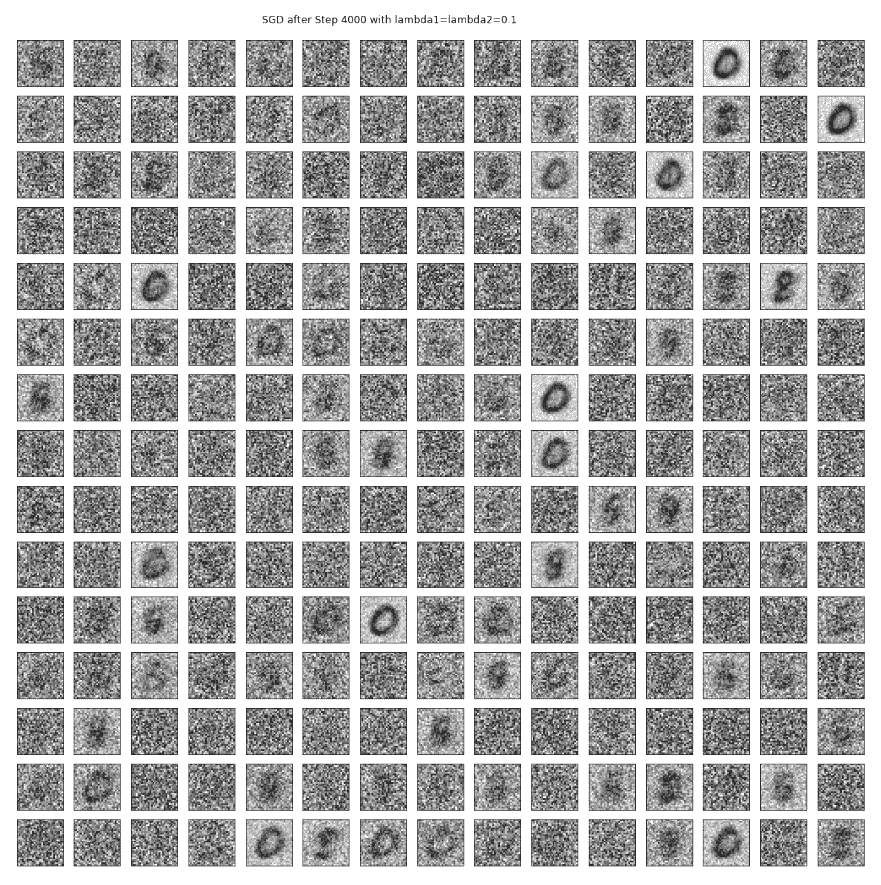pylab.figure(figsize=(8,12))
pylab.subplot(211)
pylab.plot(range(0,3001,500), atr, '.-', label='training accuracy')
pylab.plot(range(0,3001,500), av, '.-', label='validation accuracy')
pylab.xlabel('GD steps'), pylab.ylabel('Accuracy'), pylab.legend(loc='lower right')
pylab.subplot(212)
pylab.plot(range(0,3001,500), ll, '.-')
pylab.xlabel('GD steps'), pylab.ylabel('Softmax Loss')
pylab.show()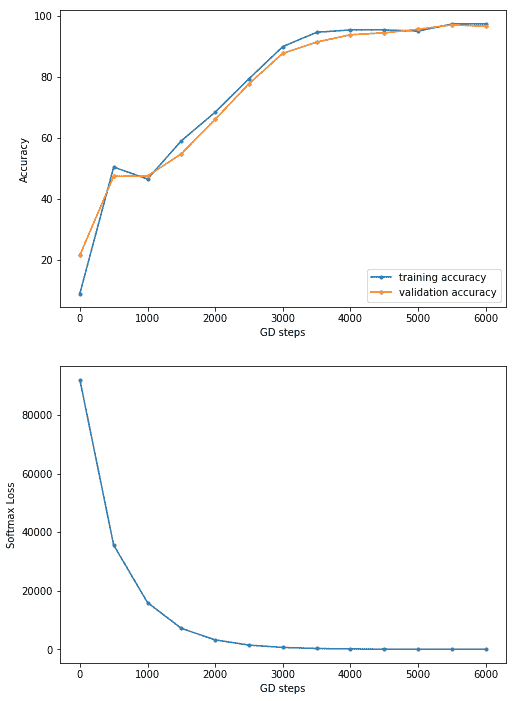# 网络可视化

# pip install pydot_ng ## install pydot_ng if not already installed
import pydot_ng as pydot
from keras.utils import plot_model
plot_model(model, to_file='../images/model.png')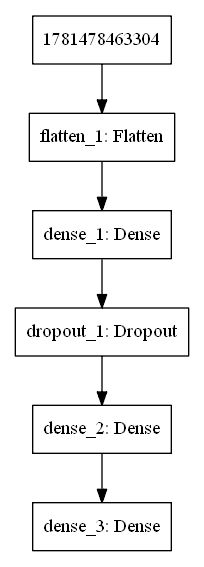# 可视化中间层中的权重

from keras.models import Modelimport matplotlib.pylab as pylabimport numpy as npW = model.get_layer('dense_1').get_weights()print(W.shape)print(W.shape)fig = pylab.figure(figsize=(20,20))fig.subplots_adjust(left=0, right=1, bottom=0, top=0.95, hspace=0.05, wspace=0.05) pylab.gray()for i in range(200):    pylab.subplot(15, 14, i+1), pylab.imshow(np.reshape(W[:, i], (28,28))), pylab.axis('off')pylab.suptitle('Dense_1 Weights (200 hidden units)', size=20)pylab.show()


# 分类 MNIST

import kerasfrom keras.models import Sequentialfrom keras.layers import Densefrom keras.utils import to_categoricalfrom keras.layers.convolutional import Conv2D # to add convolutional layersfrom keras.layers.convolutional import MaxPooling2D # to add pooling layersfrom keras.layers import Flatten # to flatten data for fully ...


# 可视化中间层

from keras.models import Model
import matplotlib.pylab as pylab
import numpy as np
intermediate_layer_model = Model(inputs=model.input, outputs=model.get_layer('conv2d_1').output)
intermediate_output = intermediate_layer_model.predict(X_train)
print(model.input.shape, intermediate_output.shape)
fig = pylab.figure(figsize=(15,15))
fig.subplots_adjust(left=0, right=1, bottom=0, top=1, hspace=0.05, wspace=0.05)
pylab.gray()
i = 1
for c in range(64):
pylab.subplot(8, 8, c+1), pylab.imshow(intermediate_output[i,:,:,c]), pylab.axis('off')
pylab.show()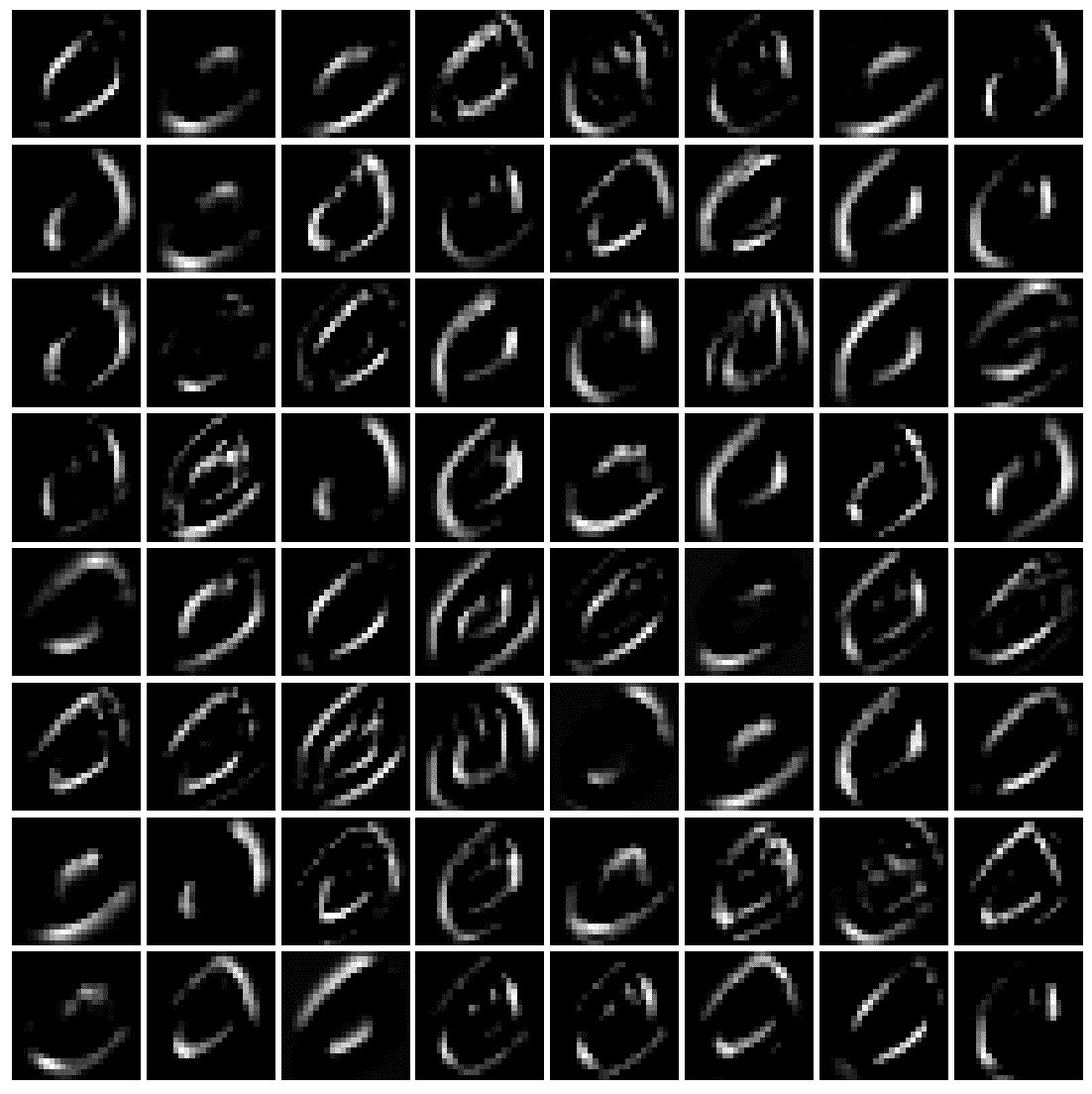i = 2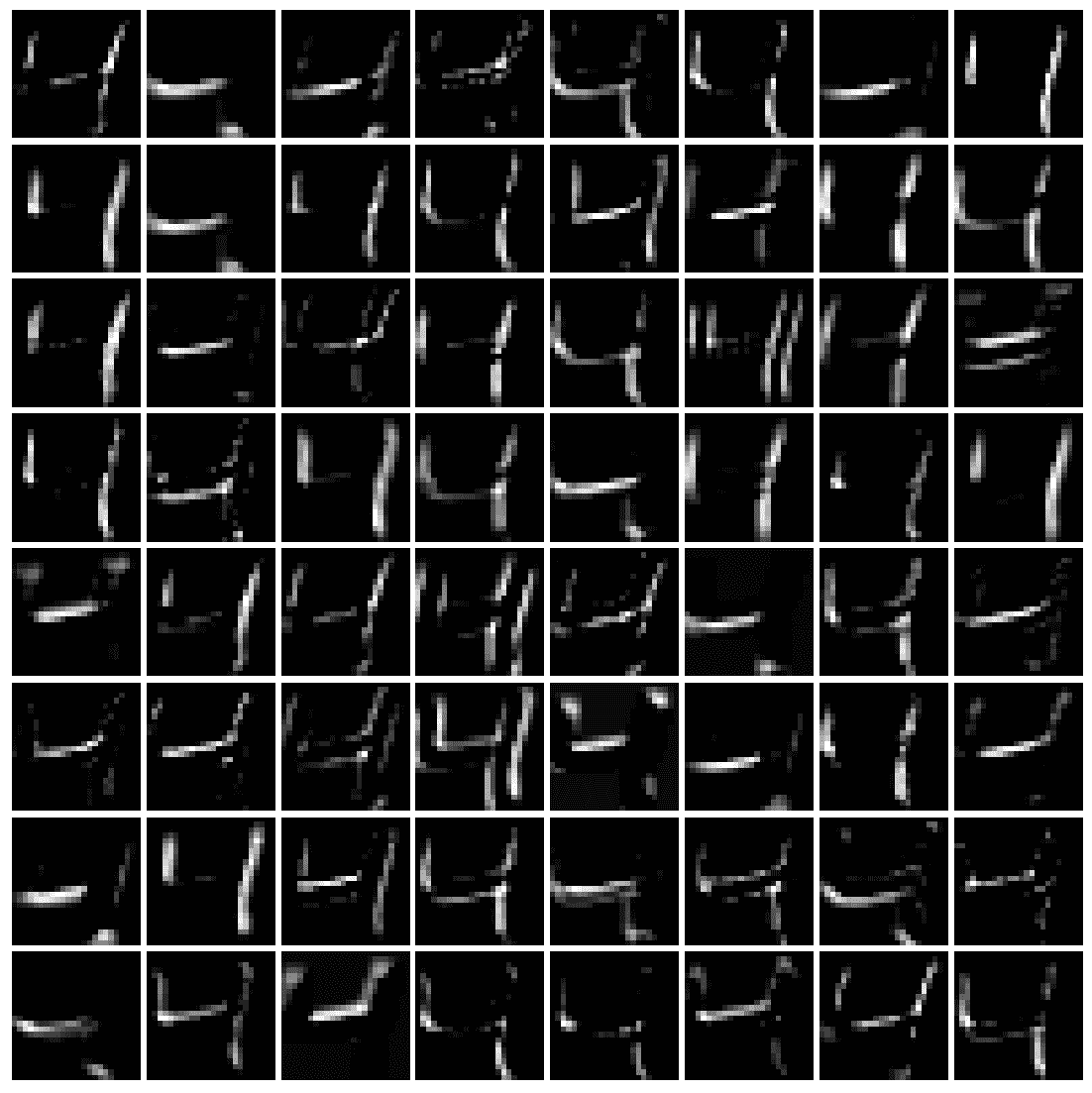# 一些流行的深度 CNN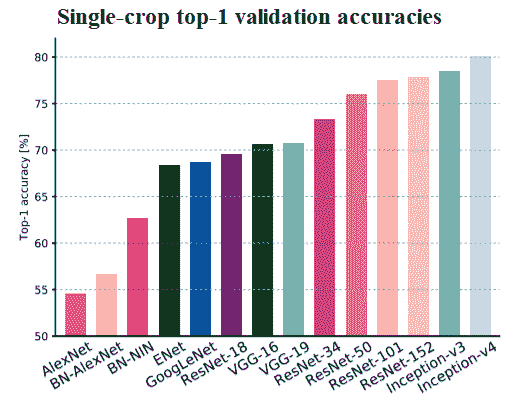# VGG-16/19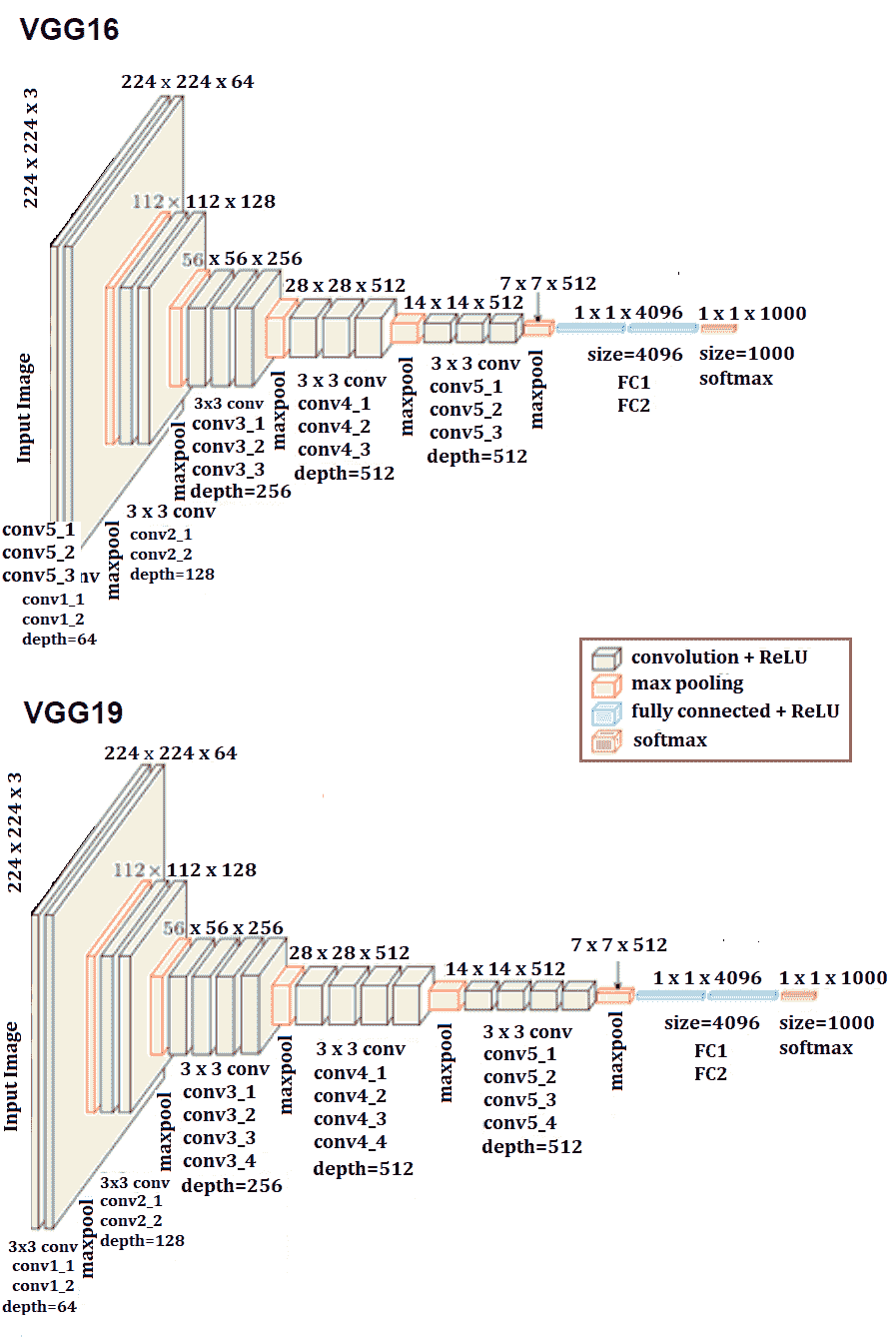# 在 Keras 中用 VGG-16 对猫/狗图像进行分类

**# 训练阶段

import os
import numpy as np
import cv2
from random import shuffle
from tqdm import tqdm # percentage bar for tasks.

# download the cats/dogs images compressed train and test datasets from here: https://www.kaggle.com/c/dogs-vs-cats/data
# unzip the train.zip images under the train folder and test.zip images under the test folder
train = './train'
test = './test'
lr = 1e-6 # learning rate
image_size = 50 # all the images will be resized to squaure images with this dimension

model_name = 'cats_dogs-{}-{}.model'.format(lr, 'conv2')

def label_image(image):
word_label = image.split('.')[-3]
if word_label == 'cat': return 0
elif word_label == 'dog': return 1

def create_training_data():
training_data = []
for image in tqdm(os.listdir(train)):
path = os.path.join(train, image)
label = label_image(image)
image = cv2.resize(image, (image_size, image_size))
training_data.append([np.array(image),np.array(label)])
shuffle(training_data)
np.save('train_data.npy', training_data)
return training_data

train_data = create_training_data()
# 100%|████████████████████████████████████████████████████████████████████████████████████████| 1100/1100 [00:00<00:00, 1133.86it/s]

train = train_data[:-5000] # 20k images for training
valid = train_data[-5000:] # 5k images for validation
X_train = np.array([i for i in train]).reshape(-1,image_size,image_size,3)
y_train = [i for i in train]
y_train = to_categorical(y_train)
print(X_train.shape, y_train.shape)

X_valid = np.array([i for i in valid]).reshape(-1,image_size,image_size,3)
y_valid = [i for i in valid]
y_valid = to_categorical(y_valid) # to one-hot encoding

num_classes = y_valid.shape # number of categories

model = VGG16(weights=None, input_shape=(image_size,image_size,3), classes=num_classes) # train VGG16 model from scratch
model.summary()

# fit the model, it's going take a long time if not run on GPU
model.fit(X_train, y_train, validation_data=(X_valid, y_valid), epochs=20, batch_size=256, verbose=2)
# evaluate the model
scores = model.evaluate(X_valid, y_valid, verbose=0)
print("Accuracy: {} \n Error: {}".format(scores, 100-scores*100))
# _______________________________________________________________
#Layer (type)                 Output Shape              Param
# =================================================================
# input_5 (InputLayer)         (None, 50, 50, 3)         0
# _________________________________________________________________
# block1_conv1 (Conv2D)        (None, 50, 50, 64)        1792
# _________________________________________________________________
# block1_conv2 (Conv2D)        (None, 50, 50, 64)        36928
# _________________________________________________________________
# block1_pool (MaxPooling2D)   (None, 25, 25, 64)        0
# _________________________________________________________________
# block2_conv1 (Conv2D)        (None, 25, 25, 128)       73856
# _________________________________________________________________
# block2_conv2 (Conv2D)        (None, 25, 25, 128)       147584
# _________________________________________________________________
# block2_pool (MaxPooling2D)   (None, 12, 12, 128)       0
# _________________________________________________________________
# block3_conv1 (Conv2D)        (None, 12, 12, 256)       295168
# _________________________________________________________________
# block3_conv2 (Conv2D)        (None, 12, 12, 256)       590080
# _________________________________________________________________
# block3_conv3 (Conv2D)        (None, 12, 12, 256)       590080
# _________________________________________________________________
# block3_pool (MaxPooling2D)   (None, 6, 6, 256)         0
# _________________________________________________________________
# block4_conv1 (Conv2D)        (None, 6, 6, 512)         1180160
# _________________________________________________________________
# block4_conv2 (Conv2D)        (None, 6, 6, 512)         2359808
# _________________________________________________________________
# block4_conv3 (Conv2D)        (None, 6, 6, 512)         2359808
# _________________________________________________________________
# block4_pool (MaxPooling2D)   (None, 3, 3, 512)         0
# _________________________________________________________________
# block5_conv1 (Conv2D)        (None, 3, 3, 512)         2359808
# _________________________________________________________________
# block5_conv2 (Conv2D)        (None, 3, 3, 512)         2359808
# _________________________________________________________________
# block5_conv3 (Conv2D)        (None, 3, 3, 512)         2359808
# _________________________________________________________________
# block5_pool (MaxPooling2D)   (None, 1, 1, 512)         0
# _________________________________________________________________
# flatten (Flatten)            (None, 512)               0
# ________________________________________________________________ *# fc1 (Dense)                  (None, 4096)              2101248* # _______________________________________________________________ *# fc2 (Dense)                  (None, 4096)              16781312* # ________________________________________________________________
# predictions (Dense)          (None, 2)                 8194
# =================================================================
# Total params: 33,605,442
# Trainable params: 33,605,442
# Non-trainable params: 0
# _________________________________________________________________
# Train on 20000 samples, validate on 5000 samples
# Epoch 1/10
# - 92s - loss: 0.6878 - acc: 0.5472 - val_loss: 0.6744 - val_acc: 0.5750
# Epoch 2/20
# - 51s - loss: 0.6529 - acc: 0.6291 - val_loss: 0.6324 - val_acc: 0.6534
# Epoch 3/20
# - 51s - loss: 0.6123 - acc: 0.6649 - val_loss: 0.6249 - val_acc: 0.6472
# Epoch 4/20
# - 51s - loss: 0.5919 - acc: 0.6842 - val_loss: 0.5902 - val_acc: 0.6828
# Epoch 5/20
# - 51s - loss: 0.5709 - acc: 0.6992 - val_loss: 0.5687 - val_acc: 0.7054
# Epoch 6/20
# - 51s - loss: 0.5564 - acc: 0.7159 - val_loss: 0.5620 - val_acc: 0.7142
# Epoch 7/20
# - 51s - loss: 0.5539 - acc: 0.7137 - val_loss: 0.5698 - val_acc: 0.6976
# Epoch 8/20
# - 51s - loss: 0.5275 - acc: 0.7371 - val_loss: 0.5402 - val_acc: 0.7298
# Epoch 9/20
# - 51s - loss: 0.5072 - acc: 0.7536 - val_loss: 0.5240 - val_acc: 0.7444
# Epoch 10/20
# - 51s - loss: 0.4880 - acc: 0.7647 - val_loss: 0.5127 - val_acc: 0.7544
# Epoch 11/20
# - 51s - loss: 0.4659 - acc: 0.7814 - val_loss: 0.5594 - val_acc: 0.7164
# Epoch 12/20
# - 51s - loss: 0.4584 - acc: 0.7813 - val_loss: 0.5689 - val_acc: 0.7124
# Epoch 13/20
# - 51s - loss: 0.4410 - acc: 0.7952 - val_loss: 0.4863 - val_acc: 0.7704
# Epoch 14/20
# - 51s - loss: 0.4295 - acc: 0.8022 - val_loss: 0.5073 - val_acc: 0.7596
# Epoch 15/20
# - 51s - loss: 0.4175 - acc: 0.8084 - val_loss: 0.4854 - val_acc: 0.7688
# Epoch 16/20
# - 51s - loss: 0.3914 - acc: 0.8259 - val_loss: 0.4743 - val_acc: 0.7794
# Epoch 17/20
# - 51s - loss: 0.3852 - acc: 0.8286 - val_loss: 0.4721 - val_acc: 0.7810
# Epoch 18/20
# - 51s - loss: 0.3692 - acc: 0.8364 - val_loss: 0.6765 - val_acc: 0.6826
# Epoch 19/20
# - 51s - loss: 0.3752 - acc: 0.8332 - val_loss: 0.4805 - val_acc: 0.7760
# Epoch 20/20
# - 51s - loss: 0.3360 - acc: 0.8586 - val_loss: 0.4711 - val_acc: 0.7838
# Accuracy: 0.7838
# Error: 21.61999999999999


intermediate_layer_model = Model(inputs=model.input, outputs=model.get_layer('block1_conv2').output)
intermediate_output = intermediate_layer_model.predict(X_train)
fig = pylab.figure(figsize=(10,10))
fig.subplots_adjust(left=0, right=1, bottom=0, top=1, hspace=0.05, wspace=0.05)
pylab.gray()
i = 3
for c in range(64):
pylab.subplot(8, 8, c+1), pylab.imshow(intermediate_output[i,:,:,c]), pylab.axis('off')
pylab.show()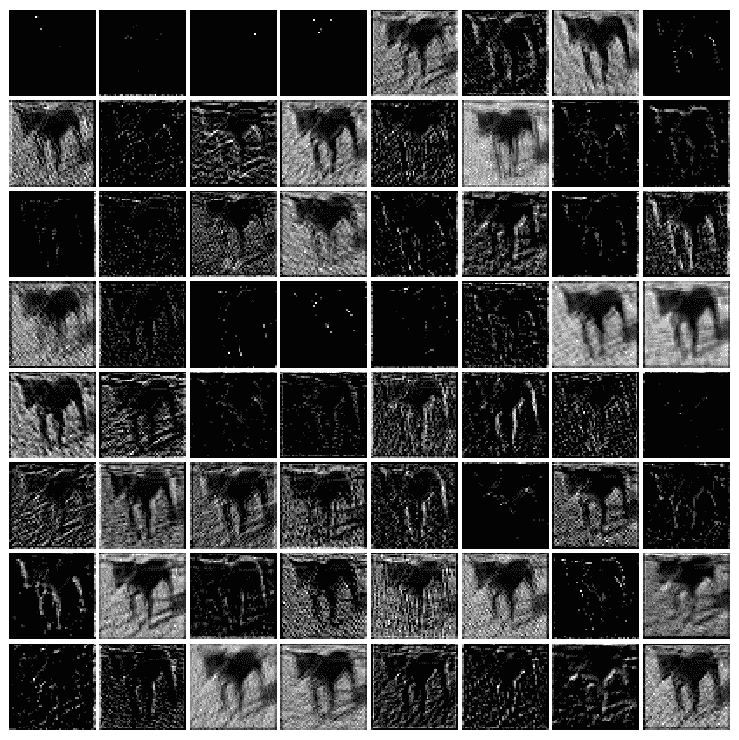intermediate_layer_model = Model(inputs=model.input, outputs=model.get_layer('block2_conv2').output)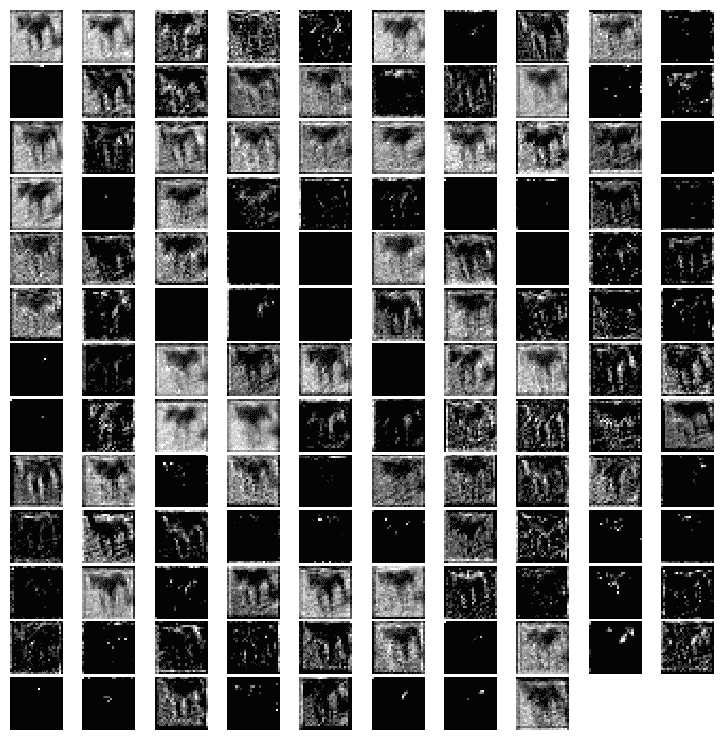# 测试（预测）阶段

test_data = process_test_data()len(test_data)X_test = np.array([i for i in test_data]).reshape(-1,IMG_SIZE,IMG_SIZE,3)probs = model.predict(X_test)probs = np.round(probs,2)pylab.figure(figsize=(20,20))for i in range(100):    pylab.subplot(10,10,i+1), pylab.imshow(X_test[i,:,:,::-1]), pylab.axis('off')    pylab.title("{}, prob={:0.2f}".format('cat' if probs[i] < 0.5 else 'dog', max(probs[i],probs[i])))pylab.show()


# 接收网

《盗梦空间》网络不断发展，并导致了网络的几个新版本的诞生。一些流行的版本是-Inception-v1、v2、v3、v4 和 Inception-ResNet。由于图像中的显著部分和信息位置可能存在巨大变化，因此为卷积运算选择正确的核大小变得非常困难。对于分布更为全局的信息，宜使用较大的核；对于分布更为局部的信息，宜使用较小的核。深层神经网络存在过度拟合和消失梯度问题。天真地堆叠大型卷积运算将招致大量费用。

inception 网络通过添加在同一级别上运行的多个大小的过滤器，解决了前面的所有问题。这导致网络变得更广而不是更深。下一个屏幕截图显示了具有降维功能的 inception 模块。它使用三种不同大小的过滤器（1 x 1、3 x 3 和 5 x 5）和额外的最大池对输入执行卷积。输出被级联并发送到下一个初始模块。为了降低成本，通过在 3 x 3 和 5 x 5 卷积之前添加额外的 1 x 1 卷积来限制输入通道的数量。利用降维初始模块，建立了神经网络结构。这就是众所周知的谷歌网初始版本 v1。该体系结构如下图所示，GoogleNet 有九个这样的初始模块线性堆叠。它有 22 层（27 层，包括池层），在上一个初始模块结束时使用全局平均池：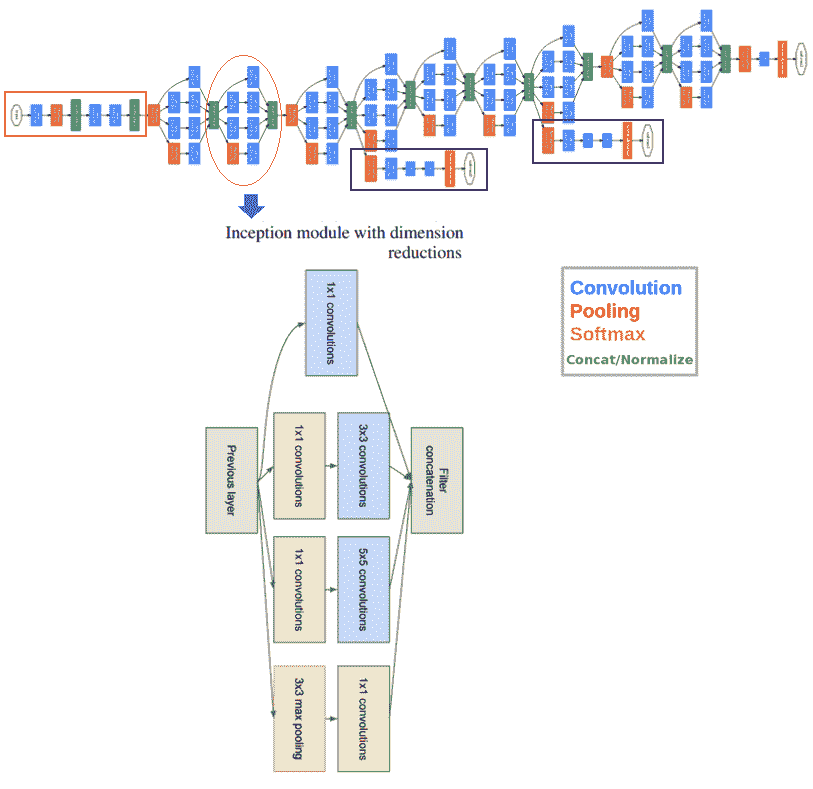# ResNet

ResNet代表剩余网络，它在网络中引入了快捷方式，我们称之为身份快捷连接。快捷连接遵循其名称，并跳过一个或多个层，从而防止堆叠层降低性能。堆叠的标识层除了简单地堆叠标识映射之外，什么都不做。。。

# 问题

1. 对于使用带有 Keras 的 FC 层对mnist数据集进行分类，编写 Python 代码片段以可视化输出层（神经网络所看到的）。
2. 对于仅使用带有 FC 层的神经网络和带有 Keras 的 CNN 对mnist数据集进行分类，我们在训练模型时直接使用测试数据集对模型进行评估。从训练图像中留出几千个图像，创建一个验证数据集，并在剩余的图像上训练模型。在培训时使用验证数据集评估模型。在培训结束时，使用所学的模型预测测试数据集的标签，并评估模型的准确性。它增加了吗？

3. 使用 VGG-16/19、Resnet-50 和 Inception。。。